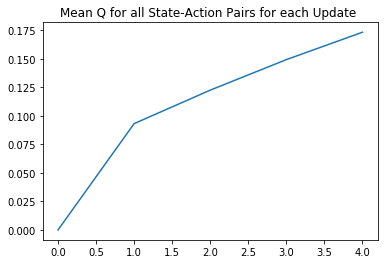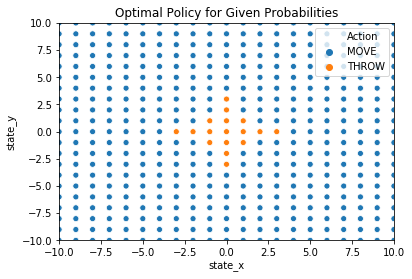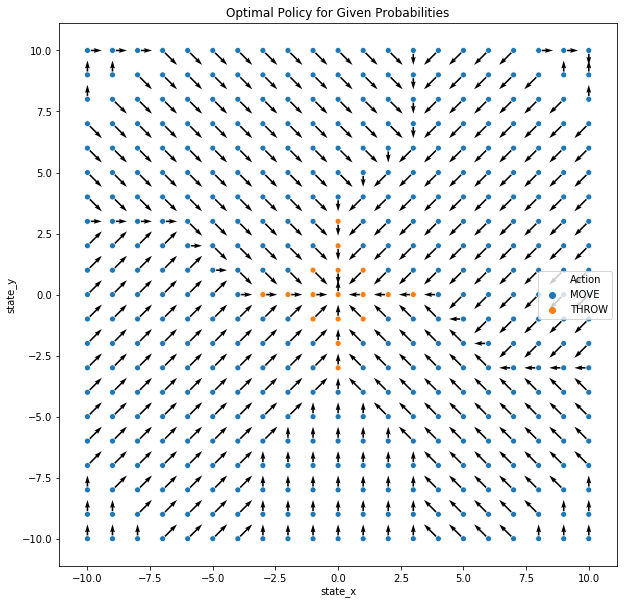# RL from Scratch Part 1.2: Finding the Optimal Policy 1

RL from scratch v1.2

# Reinforcement Learning from Scratch Part 1: Finding the Optimal Policy of an Environment Fully Defined within a Python Notebook¶

## Solving an Example Task of Throwing Paper into a Bin¶

This notebook attempts to solve a basic task of throwing paper into a bin using reinforcement learning. In this problem, we may throw from any position in the room but the probability of it is relative to the current distance from the bin and the direction in which the paper is thrown. Therefore the actions available are to throw the paper in any 360 degree direction or move to a new position to try and increase the probability that a throw made will go into the bin.

We first introduce the problem where the bin’s location is known and can be solved directly with Value-Itearation methods before showing how RL can be used similarly to find the optimal policy if the probabilities are hidden. Furthermore, we introduce the option to add control to the environment where, for example, we can punish the algorithm less for missed throws so that the algorithm will take higher risks.

Lastly, we demonstrate how the envrionment can be changed and, for example, may have walls blocking throws from certain positions.

## Part 2: Optimal Policy for Environment with Known Probabilities¶

### 2.1 Model-based Methods¶

The aim is for us to find the optimal action in each state by either throwing or moving in a given direction. Because we have known probabilities, we can actually use model-based methods and will demonstrate this first and can use value iteration to achieve this via the following formula:

\begin{equation}
Q_{k+1}(s,a) = \sum^{s’}{P(s’|s,a) (R(s,a,s’)+ γVk(s’))} \ for \ k ≥ 0
\end{equation}

where
\begin{equation}
V_k(s) = max_a \ Qk(s,a) \ for \ k>0.
\end{equation}
Value iteration starts with an arbitrary function V0 and uses the following equations to get the functions for k+1 stages to go from the functions for k stages to go (https://artint.info/html/ArtInt_227.html).

### 2.2 Initialise State-Action Pairs¶

Before applying the algorithm, we intialise each state-action value into a table. First we formthis for all throwing actions then all moving actions.

We can throw in any direction and therefore there are 360 actions for each degree starting from north as 0 clockwise to 359 degrees.

Although movement may seem simpler in that there are 8 possible actions (north, north east, east, etc) there are complications in that unlike being able to throw in any direction from any position, there are some movements that aren’t possible. For example, if we are at the edge of the room, we cannot move beyong the boundary and this needs to be accounted for. Although this could be coded nicer, I have done this manually with the if/elif statements shown that skips the row if the position and movement is not possible.

In :
#Define Q(s,a) table by all possible states and THROW actions initialised to 0
Q_table = pd.DataFrame()
for z in range(0,360):
throw_direction = int(z)
for i in range(0,21):
state_x = int(-10 + i)
for j in range(0,21):
state_y = int(-10 + j)
reward = 0
Q = pd.DataFrame({'throw_dir':throw_direction,'move_dir':"none",'state_x':state_x,'state_y':state_y,'Q':0, 'reward': reward}, index = )
Q_table = Q_table.append(Q)
Q_table = Q_table.reset_index(drop=True)
print("Q table 1 initialised")

Q table 1 initialised

Out:
throw_dir move_dir state_x state_y Q reward
0 0 none -10 -10 0 0
1 0 none -10 -9 0 0
2 0 none -10 -8 0 0
3 0 none -10 -7 0 0
4 0 none -10 -6 0 0
In :
#Define Q(s,a) table by all possible states and MOVE actions initialised to 0

for x in range(0,21):
state_x = int(-10 + x)
for y in range(0,21):
state_y = int(-10 + y)
for m in range(0,8):
move_dir = int(m)

# skip impossible moves starting with 4 corners then edges
if((state_x==10)&(state_y==10)&(move_dir==0)):
continue
elif((state_x==10)&(state_y==10)&(move_dir==2)):
continue

elif((state_x==10)&(state_y==-10)&(move_dir==2)):
continue
elif((state_x==10)&(state_y==-10)&(move_dir==4)):
continue

elif((state_x==-10)&(state_y==-10)&(move_dir==4)):
continue
elif((state_x==-10)&(state_y==-10)&(move_dir==6)):
continue

elif((state_x==-10)&(state_y==10)&(move_dir==6)):
continue
elif((state_x==-10)&(state_y==10)&(move_dir==0)):
continue

elif((state_x==10) & (move_dir == 1)):
continue
elif((state_x==10) & (move_dir == 2)):
continue
elif((state_x==10) & (move_dir == 3)):
continue

elif((state_x==-10) & (move_dir == 5)):
continue
elif((state_x==-10) & (move_dir == 6)):
continue
elif((state_x==-10) & (move_dir == 7)):
continue

elif((state_y==10) & (move_dir == 1)):
continue
elif((state_y==10) & (move_dir == 0)):
continue
elif((state_y==10) & (move_dir == 7)):
continue

elif((state_y==-10) & (move_dir == 3)):
continue
elif((state_y==-10) & (move_dir == 4)):
continue
elif((state_y==-10) & (move_dir == 5)):
continue

else:
reward = 0
Q = pd.DataFrame({'throw_dir':"none",'move_dir':move_dir,'state_x':state_x,'state_y':state_y,'Q':0, 'reward': reward}, index = )
Q_table = Q_table.append(Q)
Q_table = Q_table.reset_index(drop=True)
print("Q table 2 initialised")
Q_table.tail()

Q table 2 initialised

Out:
throw_dir move_dir state_x state_y Q reward
162035 none 6 10 9 0 0
162036 none 7 10 9 0 0
162037 none 4 10 10 0 0
162038 none 5 10 10 0 0
162039 none 6 10 10 0 0
In :
Q_table[(Q_table['state_x']==-10) &(Q_table['throw_dir']=="none")].head(5)

Out:
throw_dir move_dir state_x state_y Q reward
158760 none 0 -10 -10 0 0
158761 none 1 -10 -10 0 0
158762 none 2 -10 -10 0 0
158763 none 0 -10 -9 0 0
158764 none 1 -10 -9 0 0

### 2.3 Value-Iteration Optimal Policy¶

We start by initialising V(s) for all states, calculate the Q(s,a) matrix from this then update V(s) accordingly. This is repeated back and forth until the results converge.

Next, we calculate the probability of each state-action pair using the function introduced previously if a thrown action or simply 1 if a move action.

We are now ready to apply the Value-Iteration and introduce the two parameters gamma and the number of iterations/repeats. Gamma will effect what our algorithm values more important whether it be short of long term rewards and a value close to 1 will value long term rewards more.

“Different values of gamma may produce different policies. Lower gamma values will put more weight on short-term gains, whereas higher gamma values will put more weight towards long-term gains. Asymptotically, the closer gamma is to 1, the closer the policy will be to one that optimizes the gains over infinite time. On the other hand, value iteration will be slower to converge.

The best gamma depends on your domain. Sometimes it makes sense to look for short term gains (e.g. money gained sooner is actually more valuable than the same amount earned later), other times you want to look as far ahead as you can. And i would say that for a given MDP, there is probably a point (for high values of gamma) where the optimal policies will stabilize (no longer change when you increase gamma even more).” https://stats.stackexchange.com/questions/137590/mdp-value-iteration-choosing-gamma

The number of iterations required to converge depends entirely on the scale of the problem, we will simply try some reasonable values and then observe the results after to find the optimal value.

In :
Q_table_VI = Q_table.copy()

In :
Q_table_VI['V'] = 0

In :
bin_x = 0
bin_y = 0

prob_list = pd.DataFrame()
for n,action in enumerate(Q_table_VI['throw_dir']):
# Guarantee 100% probability if movement
if(action == "none"):
prob = 1
# Calculate if thrown
else:
prob = probability(bin_x, bin_y, Q_table_VI['state_x'][n], Q_table_VI['state_y'][n], action)
prob_list = prob_list.append(pd.DataFrame({'prob':prob}, index = [n] ))
prob_list = prob_list.reset_index(drop=True)
Q_table_VI['prob'] = prob_list['prob']

In :
Q_table_VI.head(5)

Out:
throw_dir move_dir state_x state_y Q reward V prob
0 0 none -10 -10 0 0 0 0.0
1 0 none -10 -9 0 0 0 0.0
2 0 none -10 -8 0 0 0 0.0
3 0 none -10 -7 0 0 0 0.0
4 0 none -10 -6 0 0 0 0.0
In :
Q_table_VI[ (Q_table_VI['state_x']==-1) & (Q_table_VI['state_y']==-1) & (Q_table_VI['throw_dir']==45)]

Out:
throw_dir move_dir state_x state_y Q reward V prob
17838 40 none -1 -1 0 0 0 0.8

#### Extra Code Features: Tracking loop progress and run-time¶

To improve our code, we introduce two useful tools for keep track of the run time. First, we import ‘time’ and then use this to calculate how long the Value Iteration algorithm thats to run for the given inputs.

Secondly, which I have found extremely useful for algorithms that take more than a few minutes to run, is to introduce a simple way of tracking the current progress. In short, we print the current iteration and clear this output after each stage using the second import. More info can be found here: https://www.philiposbornedata.com/2018/06/28/the-simplest-cleanest-method-for-tracking-a-for-loops-progress-and-expected-run-time-in-python-notebooks/

In :
import time
from IPython.display import clear_output

In :
input_table = Q_table_VI.copy()
gamma = 0.8
num_repeats = 5

start_time = time.time()

output_metric_table = pd.DataFrame()
# Repeat until converges
for repeats in range(0,num_repeats):
clear_output(wait=True)
state_sub_full = pd.DataFrame()

output_metric_table = output_metric_table.append(pd.DataFrame({'mean_Q':input_table['Q'].mean(),
'sum_Q': input_table['Q'].sum(),
'mean_V':input_table[['state_x', 'state_y','V']].drop_duplicates(['state_x', 'state_y', 'V'])['V'].mean(),
'sum_V': input_table[['state_x', 'state_y','V']].drop_duplicates(['state_x', 'state_y', 'V'])['V'].sum()}, index = [repeats]))

# Iterate over all states defined by max - min of x times by max - min of y
for x in range(0,21):
state_x = -10 + x
for y in range(0,21):
state_y = -10 + y

state_sub = input_table[ (input_table['state_x']==state_x) & (input_table['state_y']==state_y)]
Q_sub_list = pd.DataFrame()
for n, action in state_sub.iterrows():
# Move action update Q
if(action['throw_dir'] == "none"):
move_direction = action['move_dir']
#Map this to actual direction and find V(s) for next state
if(move_direction == 0):
move_x = 0
move_y = 1
elif(move_direction == 1):
move_x = 1
move_y = 1
elif(move_direction == 2):
move_x = 1
move_y = 0
elif(move_direction == 3):
move_x = 1
move_y = -1
elif(move_direction == 4):
move_x = 0
move_y = -1
elif(move_direction == 5):
move_x = -1
move_y = -1
elif(move_direction == 6):
move_x = -1
move_y = 0
elif(move_direction == 7):
move_x = -1
move_y = 1
Q = 1*(action['reward'] + gamma*max(input_table[ (input_table['state_x']==int(state_x+move_x)) & (input_table['state_y']==int(state_y+move_y))]['V']) )
# Throw update Q +1 if sucessful throw or -1 if failed
else:
Q = (action['prob']*(action['reward'] + gamma*1)) +  ((1-action['prob'])*(action['reward'] + gamma*-1))
Q_sub_list = Q_sub_list.append(pd.DataFrame({'Q':Q}, index = [n]))
state_sub['Q'] = Q_sub_list['Q']
state_sub['V'] = max(state_sub['Q'])
state_sub_full = state_sub_full.append(state_sub)

input_table = state_sub_full.copy()
print("Repeats completed: ", np.round((repeats+1)/num_repeats,2)*100, "%")

end_time = time.time()

print("total time taken this loop: ", np.round((end_time - start_time)/60,2), " minutes")

Repeats completed:  100.0 %
total time taken this loop:  11.32  minutes


#### Analysing Value-Iteration Output¶

We therefore have our output table that shows the quality of each state-action pair and the corresponding V value.

First, we need to conisder whether this has converged to the optimal value and can plot the mean Q values for each update. Clearly after just 10 iterations this has not converged and will need to increase this to a suitable value. It took 20 miinutes to run for 10 iterations and so we can assume that it take approximately 2 per iteration.

In :
state_sub_full.head(3)

Out:
throw_dir move_dir state_x state_y Q reward V prob
0 0 none -10 -10 -0.8 0 0.0 0.0
441 1 none -10 -10 -0.8 0 0.0 0.0
882 2 none -10 -10 -0.8 0 0.0 0.0
In :
state_sub_full[ (state_sub_full['state_x']==-4) & (state_sub_full['state_y']==-4) & (state_sub_full['Q']== max(state_sub_full[ (state_sub_full['state_x']==-4) & (state_sub_full['state_y']==-4)]['Q']))]

Out:
throw_dir move_dir state_x state_y Q reward V prob
159717 none 1 -4 -4 0.32768 0 0.32768 1.0
In :
output_metric_table

Out:
mean_Q sum_Q mean_V sum_V
0 0.000000 0.000000 0.000000 0.000000
1 -0.695966 -112774.353625 0.093130 41.070179
2 -0.694344 -112511.504479 0.122433 53.992804
3 -0.693834 -112428.799682 0.149159 65.779058
4 -0.693368 -112353.367654 0.173167 76.366585
In :
plt.plot(range(0,len(output_metric_table)), output_metric_table['mean_V'])
plt.title("Mean Q for all State-Action Pairs for each Update ")
plt.show()#### Finding the Optimal Policy for Given Results¶

Although we know this hasn’t fully converged yet, if we assume it has for now we can begin to analyse the results to find the optimal action in any given state. The optimal action is the one that has the highest Q value for th given state and is found for each state in the cell below.

In :
Q_table_VI_3 = state_sub_full.copy()

In :
optimal_action_list = pd.DataFrame()
for x in range(0,21):
state_x = int(-10 + x)
for y in range(0,21):
state_y = int(-10 + y)

Q_table_VI_3

optimal_action = pd.DataFrame({'state_x':state_x, 'state_y': state_y,
'move_dir': Q_table_VI_3[ (Q_table_VI_3['state_x']==state_x) & (Q_table_VI_3['state_y']==state_y) &  (Q_table_VI_3['Q'] == max(Q_table_VI_3[(Q_table_VI_3['state_x']==state_x) &
(Q_table_VI_3['state_y']==state_y)]['Q']))].reset_index(drop=True)['move_dir'],
'throw_dir': Q_table_VI_3[ (Q_table_VI_3['state_x']==state_x) & (Q_table_VI_3['state_y']==state_y) &  (Q_table_VI_3['Q'] == max(Q_table_VI_3[(Q_table_VI_3['state_x']==state_x) &
(Q_table_VI_3['state_y']==state_y)]['Q']))].reset_index(drop=True)['throw_dir']},
index = [state_y])
optimal_action_list = optimal_action_list.append(optimal_action)
optimal_action_list = optimal_action_list.reset_index(drop=True)

In :
optimal_action_list.head(5)

Out:
state_x state_y move_dir throw_dir
0 -10 -10 0 none
1 -10 -9 0 none
2 -10 -8 0 none
3 -10 -7 1 none
4 -10 -6 1 none
In :
optimal_action_list[(optimal_action_list['state_x']==-1)&(optimal_action_list['state_y']==-1)]

Out:
state_x state_y move_dir throw_dir
198 -1 -1 none 45
In :
optimal_action_list['Action'] = np.where( optimal_action_list['move_dir'] == 'none', 'THROW', 'MOVE'  )

In :
sns.scatterplot( x="state_x", y="state_y", data=optimal_action_list,  hue='Action')
plt.title("Optimal Policy for Given Probabilities")
plt.ylim([-10,10])
plt.xlim([-10,10])
plt.show()#### Improving Visualisation of Optimal Policy¶

Although the chart shows whether the optimal action is either a throw or move it doesn’t show us which direction these are in. Therefore, we will map each optimal action to a vector of u and v and use these to create a quiver plot (https://matplotlib.org/api/_as_gen/matplotlib.axes.Axes.quiver.html).

First, we map the move direction to its x and y components and set the actions which are throwing (currently labelled as “none”) in column to a very large negative integer so we do not have issues when we want to scale the values in the column by a factor. If we didnt do this we would recieve an error as we would be trying to divide a string element by a number and this isn’t possible. We repeat this for the throw direction column as well.

We then define the scale of the arrows and use this to define the horizontal component labelled u. For movement actions, we simply multiply the movement in the x direction by this factor and for the throw direction we either move 1 unit left or right (accounting for no horizontal movement for 0 or 180 degrees and no vertical movement at 90 or 270 degrees).

The horizontal component is then used to calculate the vertical component with some basic trigonometry where we again account for certain angles that would cause errors in the calculations.

In :
optimal_action_list['move_x'] = np.where(optimal_action_list['move_dir'] == 0, int(0),
np.where(optimal_action_list['move_dir'] == 1, int(1),
np.where(optimal_action_list['move_dir'] == 2, int(1),
np.where(optimal_action_list['move_dir'] == 3, int(1),
np.where(optimal_action_list['move_dir'] == 4, int(0),
np.where(optimal_action_list['move_dir'] == 5, int(-1),
np.where(optimal_action_list['move_dir'] == 6, int(-1),
np.where(optimal_action_list['move_dir'] == 7, int(-1),
int(-1000)
))))))))
optimal_action_list['move_y'] = np.where(optimal_action_list['move_dir'] == 0, int(1),
np.where(optimal_action_list['move_dir'] == 1, int(1),
np.where(optimal_action_list['move_dir'] == 2, int(0),
np.where(optimal_action_list['move_dir'] == 3, int(-1),
np.where(optimal_action_list['move_dir'] == 4, int(-1),
np.where(optimal_action_list['move_dir'] == 5, int(-1),
np.where(optimal_action_list['move_dir'] == 6, int(0),
np.where(optimal_action_list['move_dir'] == 7, int(1),
int(-1000)
))))))))
optimal_action_list['throw_dir_2'] = np.where(optimal_action_list['throw_dir']=="none",int(-1000), optimal_action_list['throw_dir'])

Out:
state_x state_y move_dir throw_dir Action move_x move_y throw_dir_2
0 -10 -10 0 none MOVE 0 1 -1000
1 -10 -9 0 none MOVE 0 1 -1000
2 -10 -8 0 none MOVE 0 1 -1000
3 -10 -7 1 none MOVE 1 1 -1000
4 -10 -6 1 none MOVE 1 1 -1000
5 -10 -5 1 none MOVE 1 1 -1000
6 -10 -4 1 none MOVE 1 1 -1000
7 -10 -3 1 none MOVE 1 1 -1000
8 -10 -2 1 none MOVE 1 1 -1000
9 -10 -1 1 none MOVE 1 1 -1000
In :
arrow_scale = 0.1

In :
# Define horizontal arrow component as 0.1*move direction or 0.1/-0.1 depending on throw direction
optimal_action_list['u'] = np.where(optimal_action_list['Action']=="MOVE", optimal_action_list['move_x']*arrow_scale,
np.where(optimal_action_list['throw_dir_2']==0, 0,np.where(optimal_action_list['throw_dir_2']==180, 0,
np.where(optimal_action_list['throw_dir_2']==90, arrow_scale ,np.where(optimal_action_list['throw_dir_2']==270, -arrow_scale,
np.where(optimal_action_list['throw_dir_2']<180, arrow_scale,-arrow_scale))))))

Out:
state_x state_y move_dir throw_dir Action move_x move_y throw_dir_2 u
0 -10 -10 0 none MOVE 0 1 -1000 0.0
1 -10 -9 0 none MOVE 0 1 -1000 0.0
2 -10 -8 0 none MOVE 0 1 -1000 0.0
3 -10 -7 1 none MOVE 1 1 -1000 0.1
4 -10 -6 1 none MOVE 1 1 -1000 0.1
In :
# Define vertical arrow component based 0.1*move direciton or +/- u*tan(throw_dir) accordingly
optimal_action_list['v'] = np.where(optimal_action_list['Action']=="MOVE", optimal_action_list['move_y']*arrow_scale,
np.where(optimal_action_list['throw_dir_2']==0, arrow_scale,np.where(optimal_action_list['throw_dir_2']==180, -arrow_scale,
np.where(optimal_action_list['throw_dir_2']==90, 0,np.where(optimal_action_list['throw_dir_2']==270, 0,

Out:
state_x state_y move_dir throw_dir Action move_x move_y throw_dir_2 u v
0 -10 -10 0 none MOVE 0 1 -1000 0.0 0.1
1 -10 -9 0 none MOVE 0 1 -1000 0.0 0.1
2 -10 -8 0 none MOVE 0 1 -1000 0.0 0.1
3 -10 -7 1 none MOVE 1 1 -1000 0.1 0.1
4 -10 -6 1 none MOVE 1 1 -1000 0.1 0.1
In :
x = optimal_action_list['state_x']
y = optimal_action_list['state_y']
u = optimal_action_list['u'].values
v = optimal_action_list['v'].values
plt.figure(figsize=(10, 10))
plt.quiver(x,y,u,v,scale=0.5,scale_units='inches')
sns.scatterplot( x="state_x", y="state_y", data=optimal_action_list,  hue='Action')
plt.title("Optimal Policy for Given Probabilities")
plt.show()##### We can combine the previous code for creating the quiver plot into one code cell¶
In :
# Create Quiver plot showing current optimal policy in one cell
arrow_scale = 0.1

Q_table_VI_3 = state_sub_full.copy()

optimal_action_list = pd.DataFrame()
for x in range(0,21):
state_x = int(-10 + x)
for y in range(0,21):
state_y = int(-10 + y)

Q_table_VI_3

optimal_action = pd.DataFrame({'state_x':state_x, 'state_y': state_y,
'move_dir': Q_table_VI_3[ (Q_table_VI_3['state_x']==state_x) & (Q_table_VI_3['state_y']==state_y) &  (Q_table_VI_3['Q'] == max(Q_table_VI_3[(Q_table_VI_3['state_x']==state_x) &
(Q_table_VI_3['state_y']==state_y)]['Q']))].reset_index(drop=True)['move_dir'],
'throw_dir': Q_table_VI_3[ (Q_table_VI_3['state_x']==state_x) & (Q_table_VI_3['state_y']==state_y) &  (Q_table_VI_3['Q'] == max(Q_table_VI_3[(Q_table_VI_3['state_x']==state_x) &
(Q_table_VI_3['state_y']==state_y)]['Q']))].reset_index(drop=True)['throw_dir']},
index = [state_y])
optimal_action_list = optimal_action_list.append(optimal_action)
optimal_action_list = optimal_action_list.reset_index(drop=True)

optimal_action_list['Action'] = np.where( optimal_action_list['move_dir'] == 'none', 'THROW', 'MOVE'  )

optimal_action_list['move_x'] = np.where(optimal_action_list['move_dir'] == 0, int(0),
np.where(optimal_action_list['move_dir'] == 1, int(1),
np.where(optimal_action_list['move_dir'] == 2, int(1),
np.where(optimal_action_list['move_dir'] == 3, int(1),
np.where(optimal_action_list['move_dir'] == 4, int(0),
np.where(optimal_action_list['move_dir'] == 5, int(-1),
np.where(optimal_action_list['move_dir'] == 6, int(-1),
np.where(optimal_action_list['move_dir'] == 7, int(-1),
int(-1000)
))))))))
optimal_action_list['move_y'] = np.where(optimal_action_list['move_dir'] == 0, int(1),
np.where(optimal_action_list['move_dir'] == 1, int(1),
np.where(optimal_action_list['move_dir'] == 2, int(0),
np.where(optimal_action_list['move_dir'] == 3, int(-1),
np.where(optimal_action_list['move_dir'] == 4, int(-1),
np.where(optimal_action_list['move_dir'] == 5, int(-1),
np.where(optimal_action_list['move_dir'] == 6, int(0),
np.where(optimal_action_list['move_dir'] == 7, int(1),
int(-1000)
))))))))
optimal_action_list['throw_dir_2'] = np.where(optimal_action_list['throw_dir']=="none",int(-1000), optimal_action_list['throw_dir'])

# Define horizontal arrow component as 0.1*move direction or 0.1/-0.1 depending on throw direction
optimal_action_list['u'] = np.where(optimal_action_list['Action']=="MOVE", optimal_action_list['move_x']*arrow_scale,
np.where(optimal_action_list['throw_dir_2']==0, 0,np.where(optimal_action_list['throw_dir_2']==180, 0,
np.where(optimal_action_list['throw_dir_2']==90, arrow_scale ,np.where(optimal_action_list['throw_dir_2']==270, -arrow_scale,
np.where(optimal_action_list['throw_dir_2']<180, arrow_scale,-arrow_scale))))))

# Define vertical arrow component based 0.1*move direciton or +/- u*tan(throw_dir) accordingly
optimal_action_list['v'] = np.where(optimal_action_list['Action']=="MOVE", optimal_action_list['move_y']*arrow_scale,
np.where(optimal_action_list['throw_dir_2']==0, arrow_scale,np.where(optimal_action_list['throw_dir_2']==180, -arrow_scale,
np.where(optimal_action_list['throw_dir_2']==90, 0,np.where(optimal_action_list['throw_dir_2']==270, 0,

x = optimal_action_list['state_x']
y = optimal_action_list['state_y']
u = optimal_action_list['u'].values
v = optimal_action_list['v'].values

#plt.figure(figsize=(10, 10))
#plt.quiver(x,y,u,v,scale=0.5,scale_units='inches')
#sns.scatterplot( x="state_x", y="state_y", data=optimal_action_list,  hue='Action')
#plt.title("Optimal Policy for Given Probabilities")
#plt.show()